# 0/5 points I Previous Answers My Compute the work done by the force F (sin(, y, in moving an obje...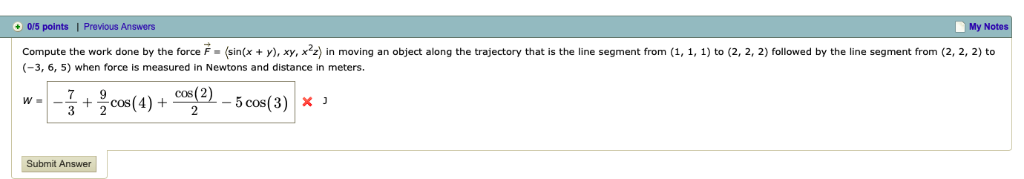0/5 points I Previous Answers My Compute the work done by the force F (sin(, y, in moving an object along the trajectory that is the line segment from (1, 1, ) to (2, 2, 2) followed by the line segment from (2,2, 2) to (-3, 6, 5) when force is measured in Newtons and distance in meters. Submit Answer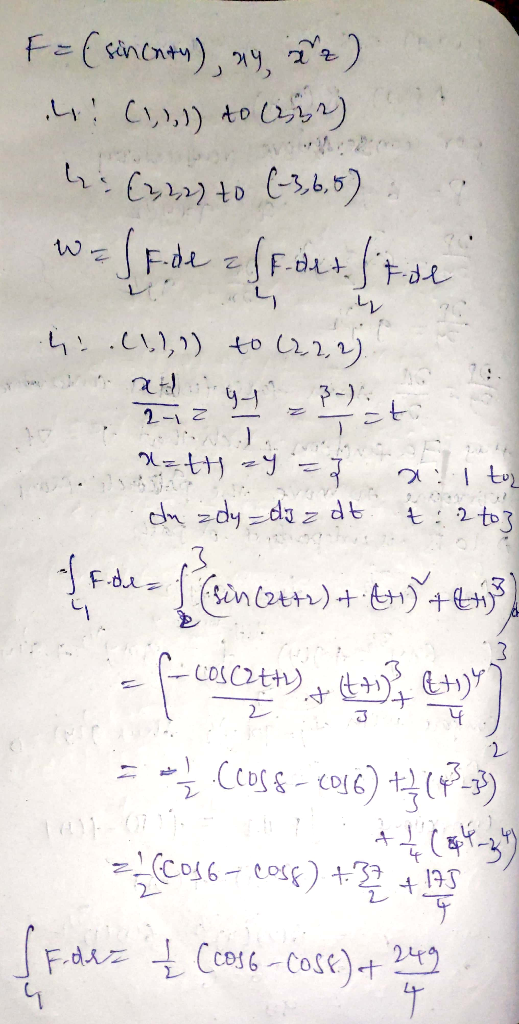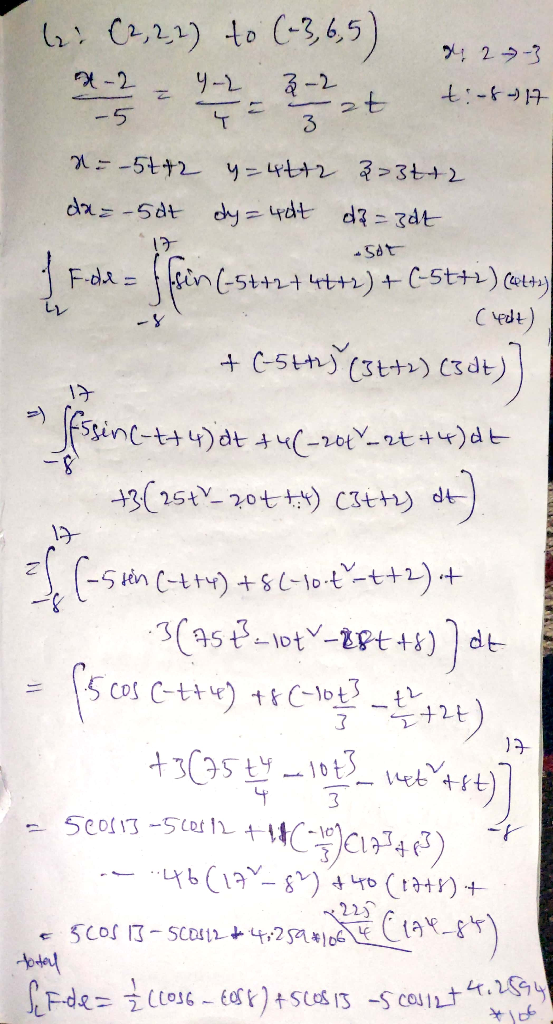If you have any questions please let me know

Thank you

##### Add Answer of: 0/5 points I Previous Answers My Compute the work done by the force F (sin(, y, in moving an obje...
Similar Homework Help Questions
• ### ху, x22 compute the work done by the torce F meters. sin х у in mov ng an o ect along the traject...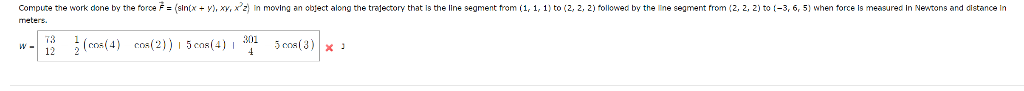ху, x22 compute the work done by the torce F meters. sin х у in mov ng an o ect along the trajectory that is the line segment from 1 1 1) to 2, ži til ed by the ine se me trom Z to 5) when or e me sure i N tons and distance in 73 1 12 2 ху, x22 compute the work done by the torce F meters. sin х у in mov ng an o...

• ### find work done (vectors)

A constant force F=-9i+2j-8k moves an object along a straight line from point (2, -2, -1) to point (-7, -8, -8).Find the work done if the distance is measured in meters and the magnitude of the force is measured in newtons.

• ### force in 3d

A constant force moves an object along a straight line from point (8, -3, 2) to point (5, -8, -5).Find the work done if the distance is measured in meters and the magnitude of the force is measured in newtons.

• ### Vectors and Work

A constant force F = -5i - 9j -3k moves an object along a straight line from point (-1, 1, -8) to point (-1, -2, 7).Find the work done if the distance is measured in meters and the magnitude of the force is measured in newtons.

• ### calc 3A constant force moves an object along a straight line from point (1, 9, 9) to point(2, 10, 5).Find the work done if the distance is measured in meters and themagnitude of the force is measured in newtons.Work =

• ### (2) If the point of application of a force F: R3 R moves along a curve C, then the work done by t...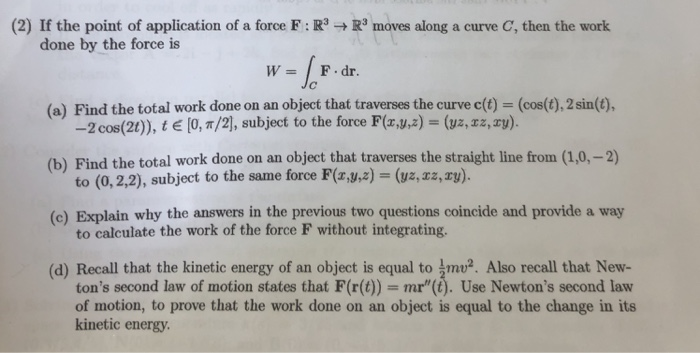all questions clearly solved please (2) If the point of application of a force F: R3 R moves along a curve C, then the work done by the force is W F.dr. (a) Find the total work done on an object that traverses the curve c(t) (cos(t), 2 sin(t), (b) Find the total work done on an object that traverses the straight line from (1,0,-2) (c) Explain why the answers in the previous two questions coincide and provide a way...

• ### 3. 0/1.25 points| Previous Answers SerPSE10 5.C.OP.051. 2/7 Submissions Used My Notes A force F (7.301...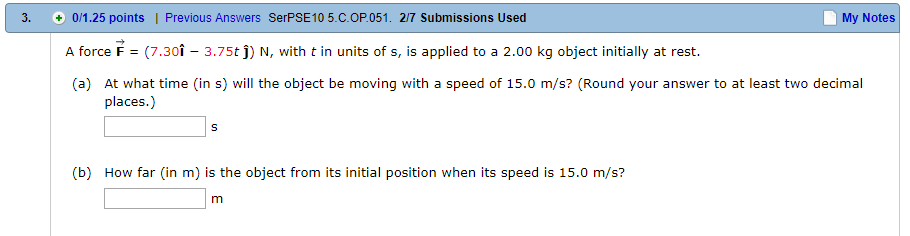3. 0/1.25 points| Previous Answers SerPSE10 5.C.OP.051. 2/7 Submissions Used My Notes A force F (7.301 3.75tj) N, with t in units of s, is applied to a 2.00 kg object initially at rest. ttime (in s) wil the object be moving with speed of is omVs? (Round your answer to at least two ceomal places (b) How far (in m) is the object from its initial position when its speed is 15.0 m/s?

• ### Please answer as many of the questions as you can, I will rate you well. Thanks :) 14. 5/14 points | Previaus Answers SCalcETB 11.11.021 Consider the following function. nx) = x sin(x), a = 0, η = 4,...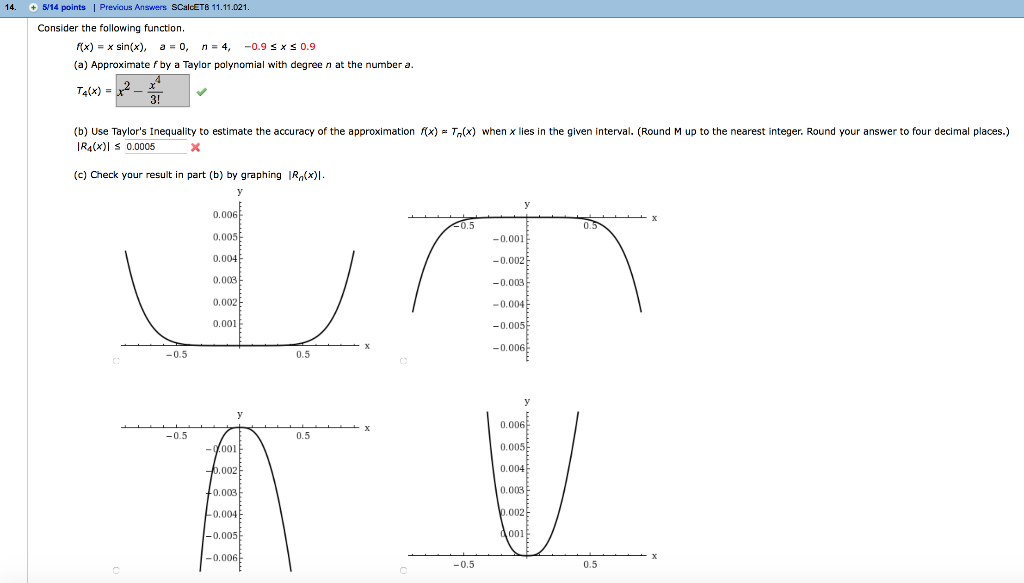Please answer as many of the questions as you can, I will rate you well. Thanks :) 14. 5/14 points | Previaus Answers SCalcETB 11.11.021 Consider the following function. nx) = x sin(x), a = 0, η = 4, -0.9 x 0.9 (a) Approximate fby a Taylor polynomial with degree n at the number a. T4(x) 3! (b Use Taylor's Inequality to estimate the accuracy of the approximation IR4(x) 0.0005 when x lies in the given interval. Round M up...

• ### force field: find the work done by F in moving object from (0,1) to (1,2) along the path from x=0 to x=1 We were una...

force field: find the work done by F in moving object from (0,1) to (1,2) along the path from x=0 to x=1 We were unable to transcribe this imagey = 1 + sin(π·r/2) Show transcribed image text

• ### 17. 0/2 points | Previous Answers My Notes A train moving at a constant speed of...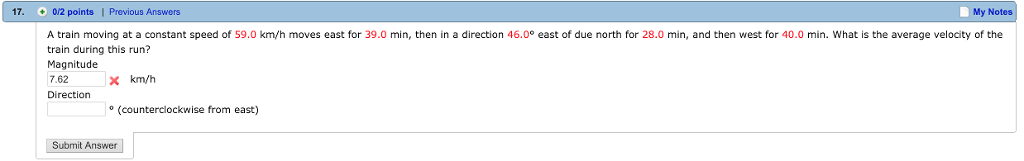17. 0/2 points | Previous Answers My Notes A train moving at a constant speed of 59.0 km/h moves east for 39.0 min, then in a direction 46.0° east of due north for 28.0 min, and then west for 40.0 min. What is the average velocity of the train during this run? Magnitude 7.62 × km/h Direction o (counterclockwise from east) Submit Answer

Free Homework App